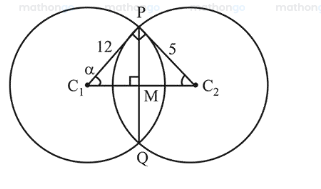# If the angle of intersection at a point where

Question:

If the angle of intersection at a point where the two circles with radii $5 \mathrm{~cm}$ and $12 \mathrm{~cm}$ intersect is $90^{\circ}$, then the length (in $\mathrm{cm}$ ) of their common chord is :

1. (1) $\frac{13}{5}$

2. (2) $\frac{120}{13}$

3. (3) $\frac{60}{13}$

4. (4) $\frac{13}{2}$

Correct Option: , 2

Solution:According to the diagram,

In $\Delta P C_{1} C_{2}, \tan \alpha=\frac{5}{12} \Rightarrow \sin \alpha=\frac{5}{13}$

In $\triangle P C_{1} M, \sin \alpha=\frac{P M}{12} \Rightarrow \frac{5}{13}=\frac{P M}{12} \Rightarrow P M=\frac{60}{13}$

In $\Delta P C_{1} M, \sin \alpha=\frac{P M}{12} \Rightarrow \frac{5}{13}=\frac{P M}{12} \Rightarrow P M=\frac{60}{13}$

Hence, length of common chord $(P Q)=\frac{120}{13}$